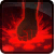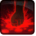# Vicious LungeConditions

Used by

## Related effects, buffs and debuffs

Please click on an effect below to view its details.

• [2s]Vicious Lunge
Malgus is preparing for a very powerful attack!

 Slot: Debuff Duration: 2s Tick rate: does not tick # occurrences: 0
• On Apply

Only when the following conditions are met:

• If difficulty is set to 16-man

Perform the following actions:

• Spell Damage
- Unknown (609) = (bool) false
- Damage Type = (int) 1
- Slot = (int) 1
- Spell Type = (int) 3
- Level Cap = (int) 0
- Amount Max = (float) 0
- Amount Min = (float) 0
- Amount Percent = (float) 0
- Threat Percent = (float) 0
- Standard Health Percent Max = (float) 0.12
- Standard Health Percent Min = (float) 0.12
- Amount Modifier Percent = (float) 0
- Coefficient = (float) 0
- Health Steal Percentage = (float) 0
• On Apply

Only when the following conditions are met:

• If difficulty is set to 8-man HM

Perform the following actions:

• Spell Damage
- Unknown (609) = (bool) false
- Damage Type = (int) 1
- Slot = (int) 1
- Spell Type = (int) 3
- Level Cap = (int) 0
- Amount Max = (float) 0
- Amount Min = (float) 0
- Amount Percent = (float) 0
- Threat Percent = (float) 0
- Standard Health Percent Max = (float) 0.12
- Standard Health Percent Min = (float) 0.12
- Amount Modifier Percent = (float) 0
- Coefficient = (float) 0
- Health Steal Percentage = (float) 0
• On Apply

Only when the following conditions are met:

• If difficulty is set to 8-man SM

Perform the following actions:

• Spell Damage
- Unknown (609) = (bool) false
- Damage Type = (int) 1
- Slot = (int) 1
- Spell Type = (int) 3
- Level Cap = (int) 0
- Amount Max = (float) 0
- Amount Min = (float) 0
- Amount Percent = (float) 0
- Threat Percent = (float) 0
- Standard Health Percent Max = (float) 0.12
- Standard Health Percent Min = (float) 0.12
- Amount Modifier Percent = (float) 0
- Coefficient = (float) 0
- Health Steal Percentage = (float) 0
• On Apply

Perform the following actions:

• Play appearance epp . sith_warrior . force_charge . cast_instant
• Teleport To Target
• Add effect #2 to TARGET from TARGET, Combat Math Only
• [0s] [not displayed]Effect #2

 Slot: Debuff Duration: 0s Tick rate: does not tick # occurrences: 0 Conditions: Can only by called by other effects
• On Apply
• [target override]

Only when the following conditions are met:

• <
• If TARGET != PRIMARY TARGET
AND
• If target is an enemy
AND
• If PRIMARY TARGET is alive
>

Perform the following actions:

• Ballistic Impulse, centered at caster, immediately knockdown, momentum = 1, 35° pitch, 0° yaw
• Add effect #3 to TARGET from TARGET, Combat Math Only
• On Apply

Perform the following actions:

• Play appearance epp . flashpoint . corellia . republic . enemy . boss . boss_3 . vicious_lunge_impact
• [1.5s]Vicious Lunge
Stunned.

 Slot: Debuff Duration: 1.5s Tick rate: does not tick # occurrences: 0 Tags: tag.​abl.​debuff.​stun Conditions: Can only by called by other effects
• On Apply

Perform the following actions:

• Stun the TARGET
• On Apply

Only when the following conditions are met:

• If difficulty is set to 16-man

Perform the following actions:

• Spell Damage
- Unknown (609) = (bool) false
- Damage Type = (int) 1
- Slot = (int) 1
- Spell Type = (int) 3
- Level Cap = (int) 0
- Amount Max = (float) 0
- Amount Min = (float) 0
- Amount Percent = (float) 0
- Threat Percent = (float) 0
- Standard Health Percent Max = (float) 0.12
- Standard Health Percent Min = (float) 0.12
- Amount Modifier Percent = (float) 0
- Coefficient = (float) 0
- Health Steal Percentage = (float) 0
• On Apply

Only when the following conditions are met:

• If difficulty is set to 8-man SM

Perform the following actions:

• Spell Damage
- Unknown (609) = (bool) false
- Damage Type = (int) 1
- Slot = (int) 1
- Spell Type = (int) 3
- Level Cap = (int) 0
- Amount Max = (float) 0
- Amount Min = (float) 0
- Amount Percent = (float) 0
- Threat Percent = (float) 0
- Standard Health Percent Max = (float) 0.12
- Standard Health Percent Min = (float) 0.12
- Amount Modifier Percent = (float) 0
- Coefficient = (float) 0
- Health Steal Percentage = (float) 0
• On Apply

Only when the following conditions are met:

• If difficulty is set to 8-man HM

Perform the following actions:

• Spell Damage
- Unknown (609) = (bool) false
- Damage Type = (int) 1
- Slot = (int) 1
- Spell Type = (int) 3
- Level Cap = (int) 0
- Amount Max = (float) 0
- Amount Min = (float) 0
- Amount Percent = (float) 0
- Threat Percent = (float) 0
- Standard Health Percent Max = (float) 0.12
- Standard Health Percent Min = (float) 0.12
- Amount Modifier Percent = (float) 0
- Coefficient = (float) 0
- Health Steal Percentage = (float) 0